# RS Aggarwal Solutions for Class 7 Maths Chapter 22 Bar Graphs

RS Aggarwal Solutions for Class 7 Maths Chapter 22 – Bar Graphs are provided here. To cover the entire syllabus in Maths, the RS Aggarwal is an essential material as it offers a wide range of questions that test the students’ understanding of concepts. Our expert personnel have solved the problems step by step with neat explanations. Students who aspire to score good marks in Maths, then refer RS Aggarwal Class 7 Solutions.

Expert tutors at BYJU’S have prepared the RS Aggarwal Solutions for Class 7 Maths Chapter 22, which contains all exercises. Students gain more knowledge by referring to RS Aggarwal Solutions. Download pdf of Class 7 Chapter 22 in their respective links.

## Download PDF of RS Aggarwal Solutions for Class 7 Maths Chapter 22 – Bar Graphs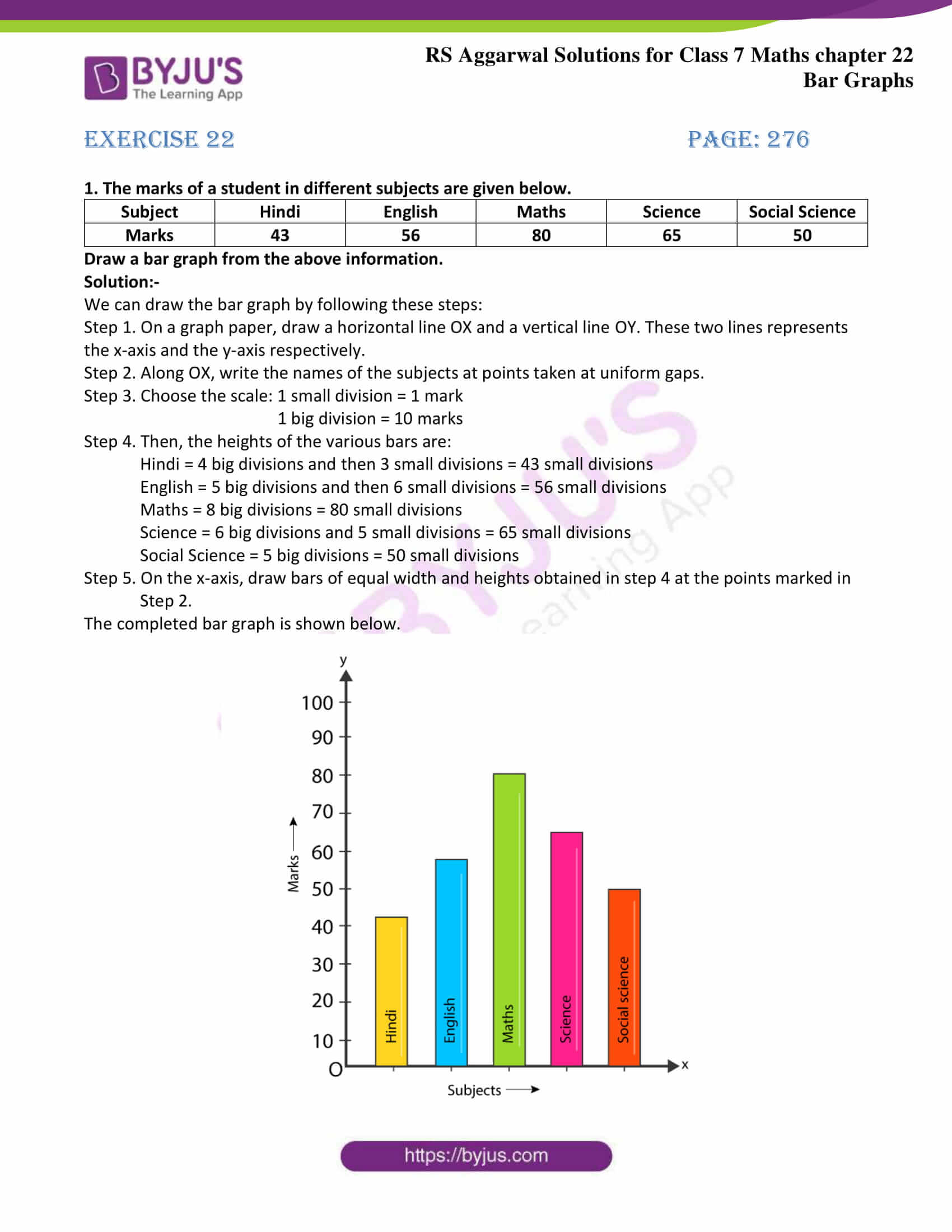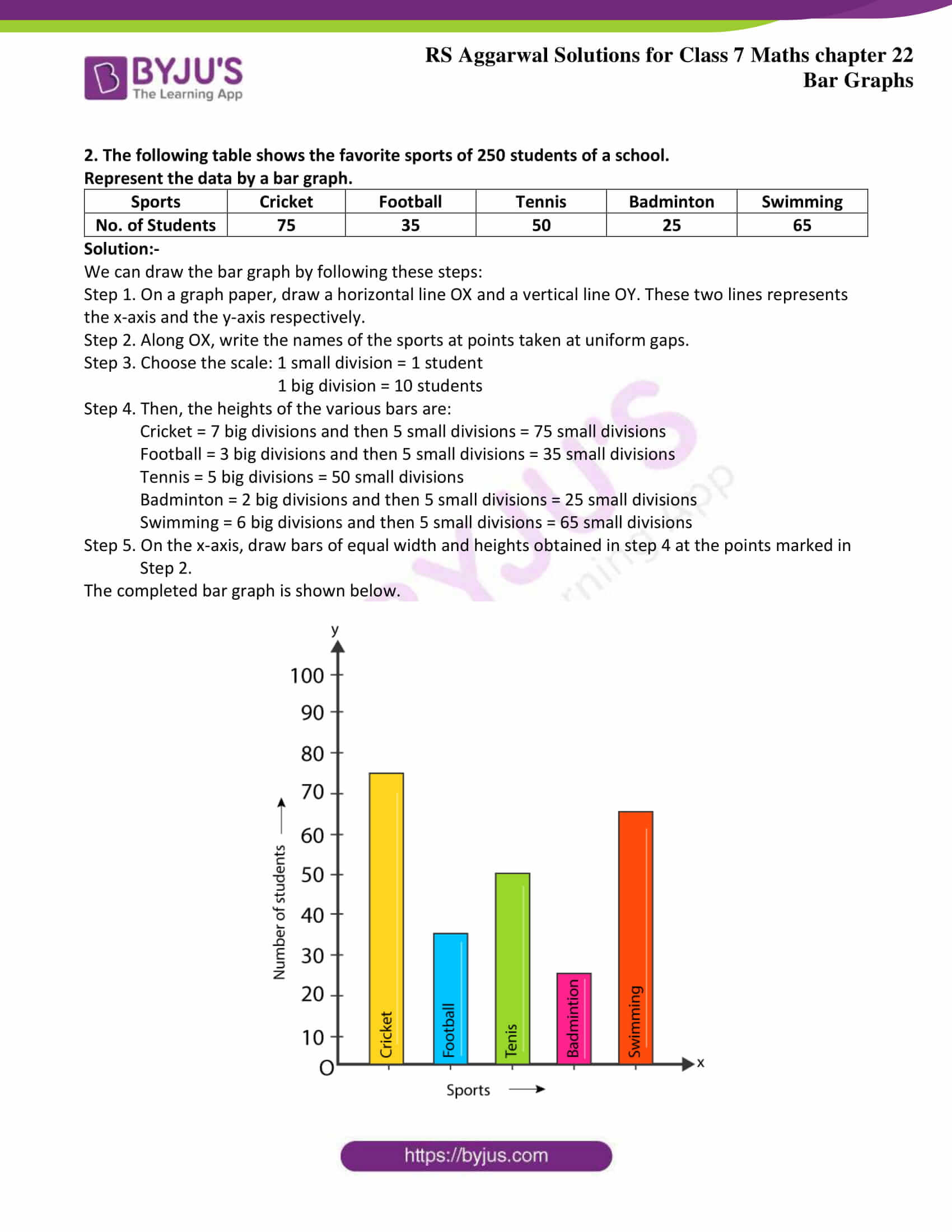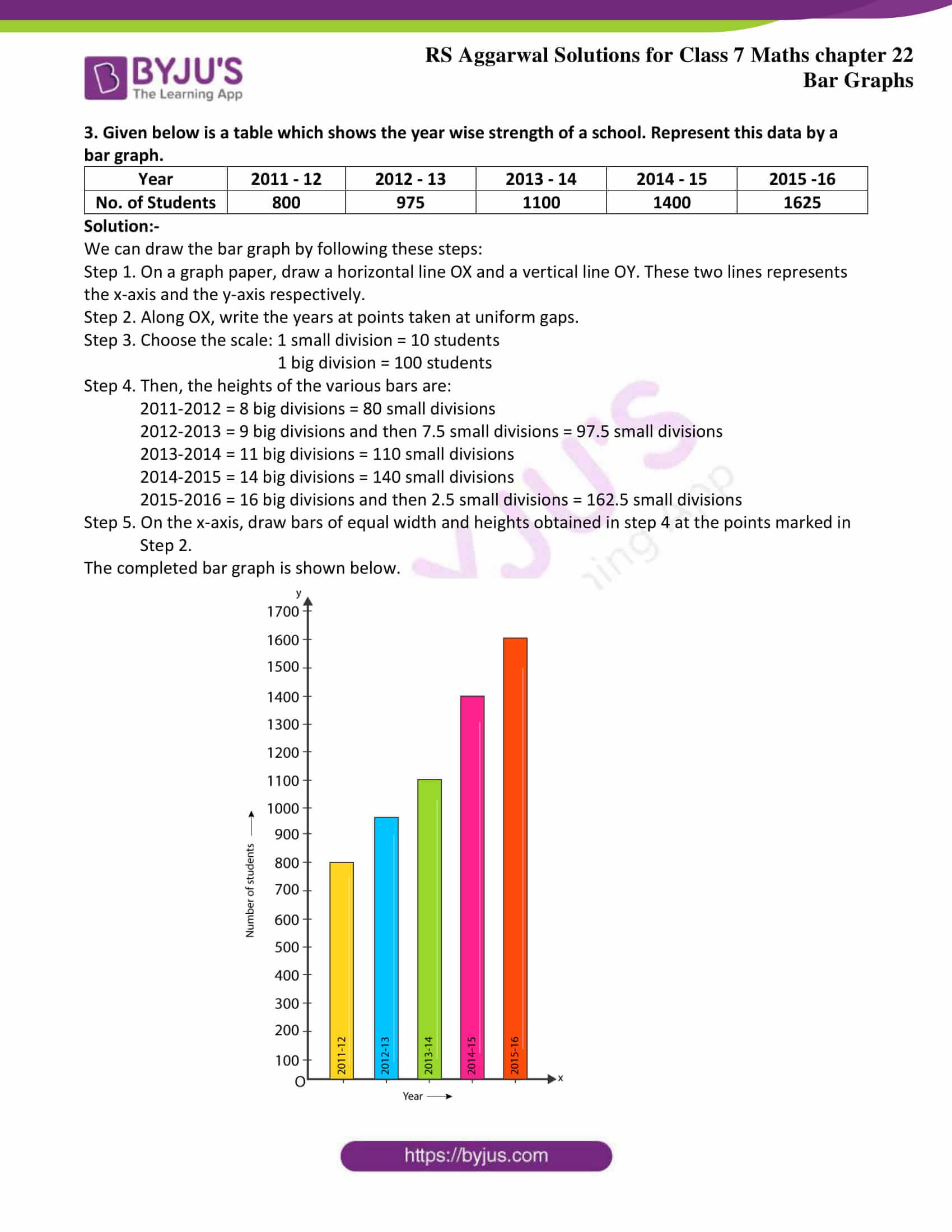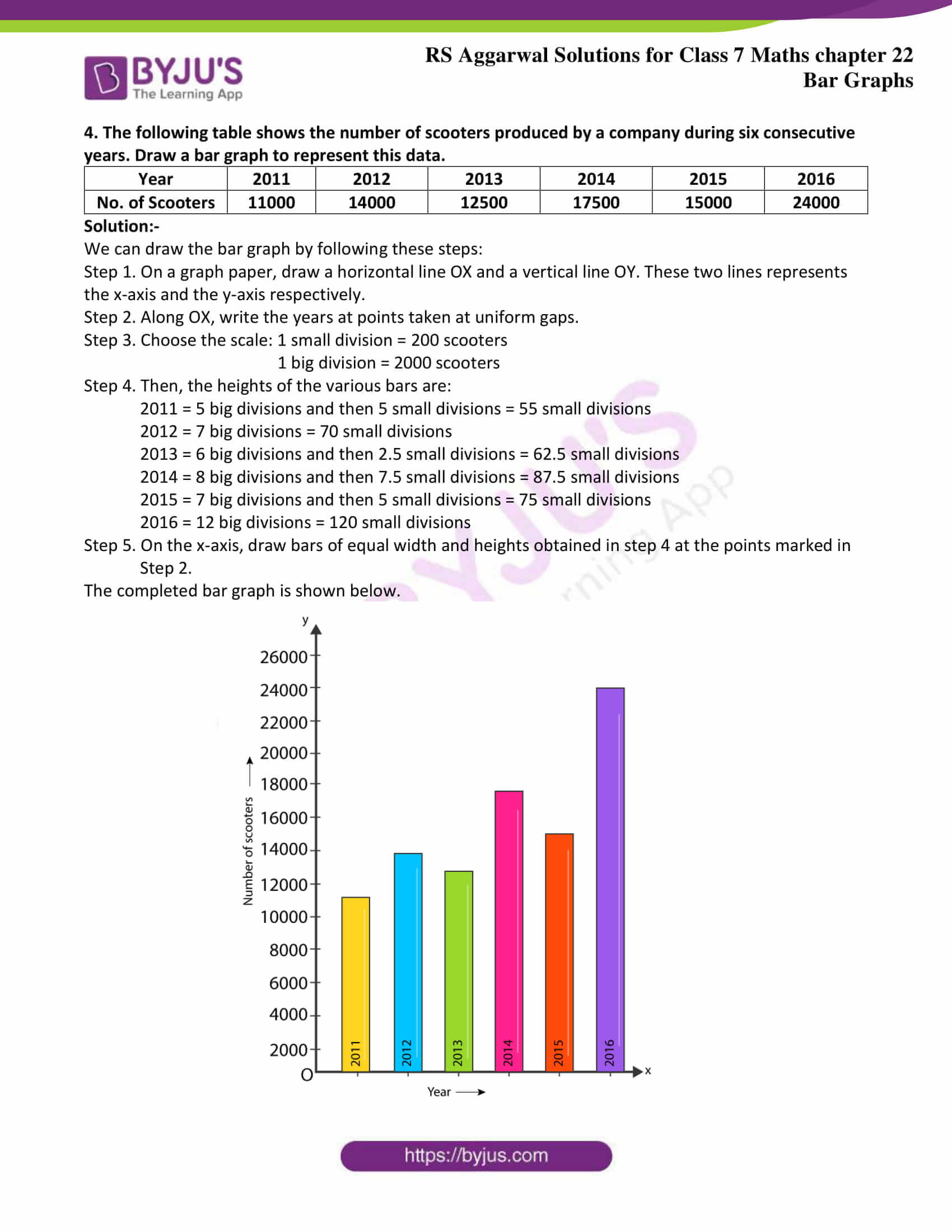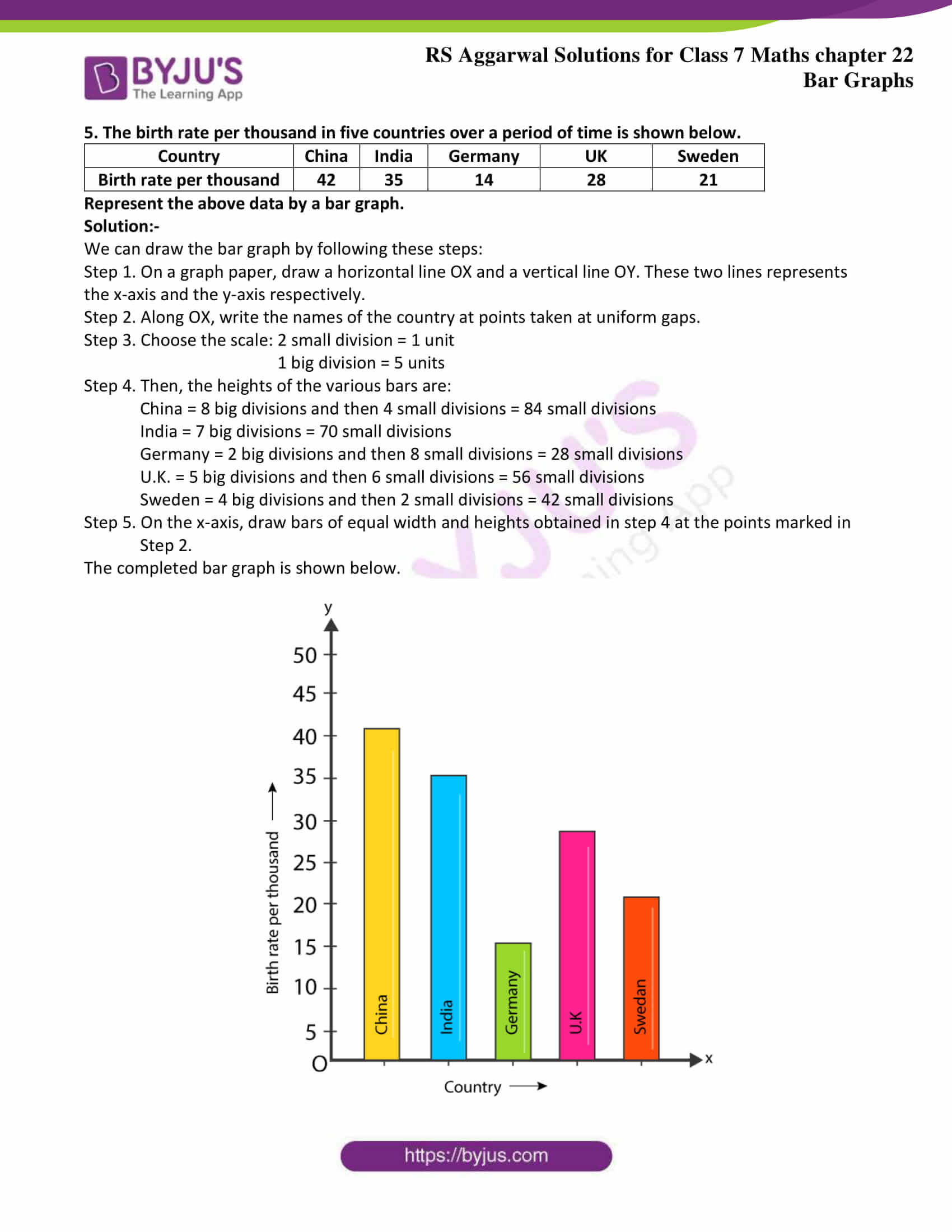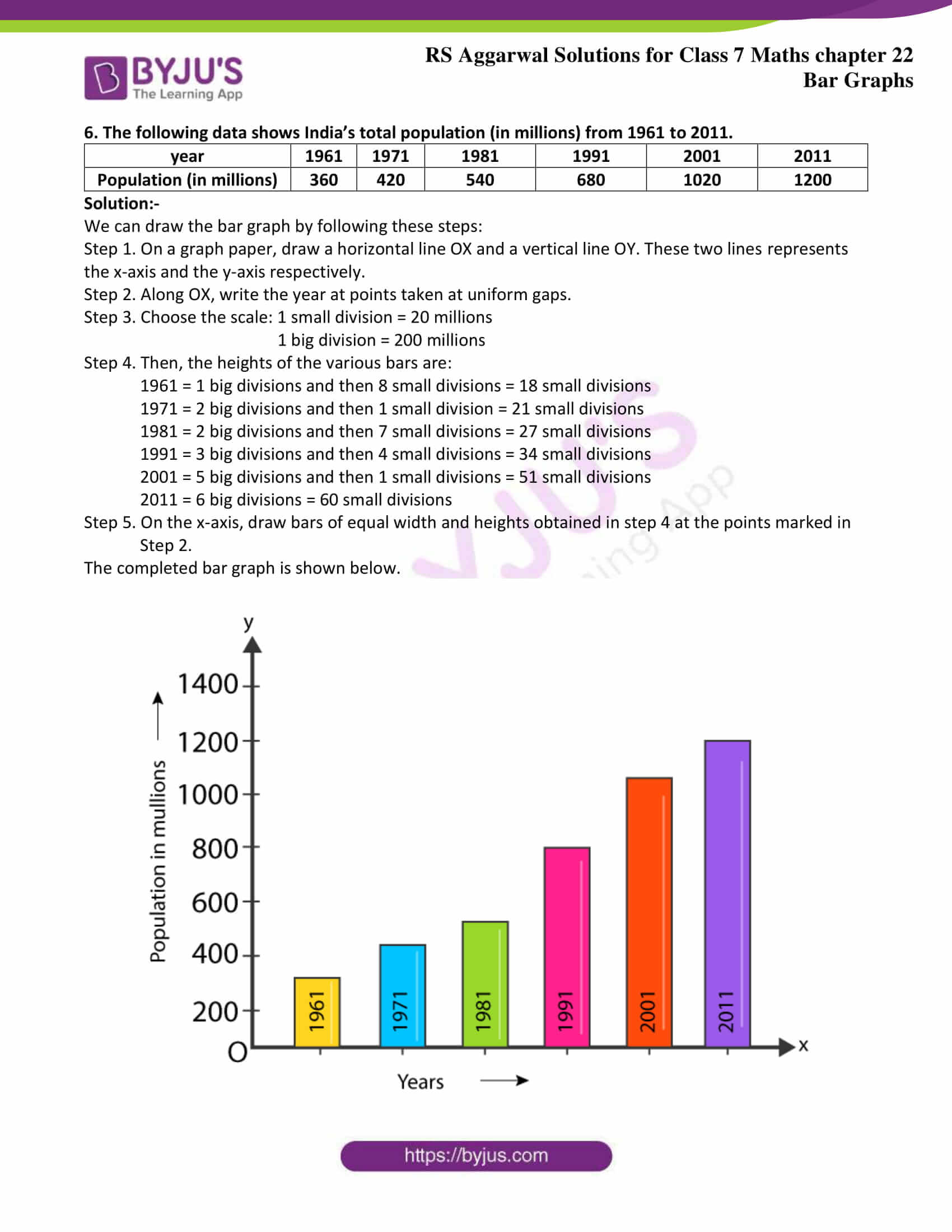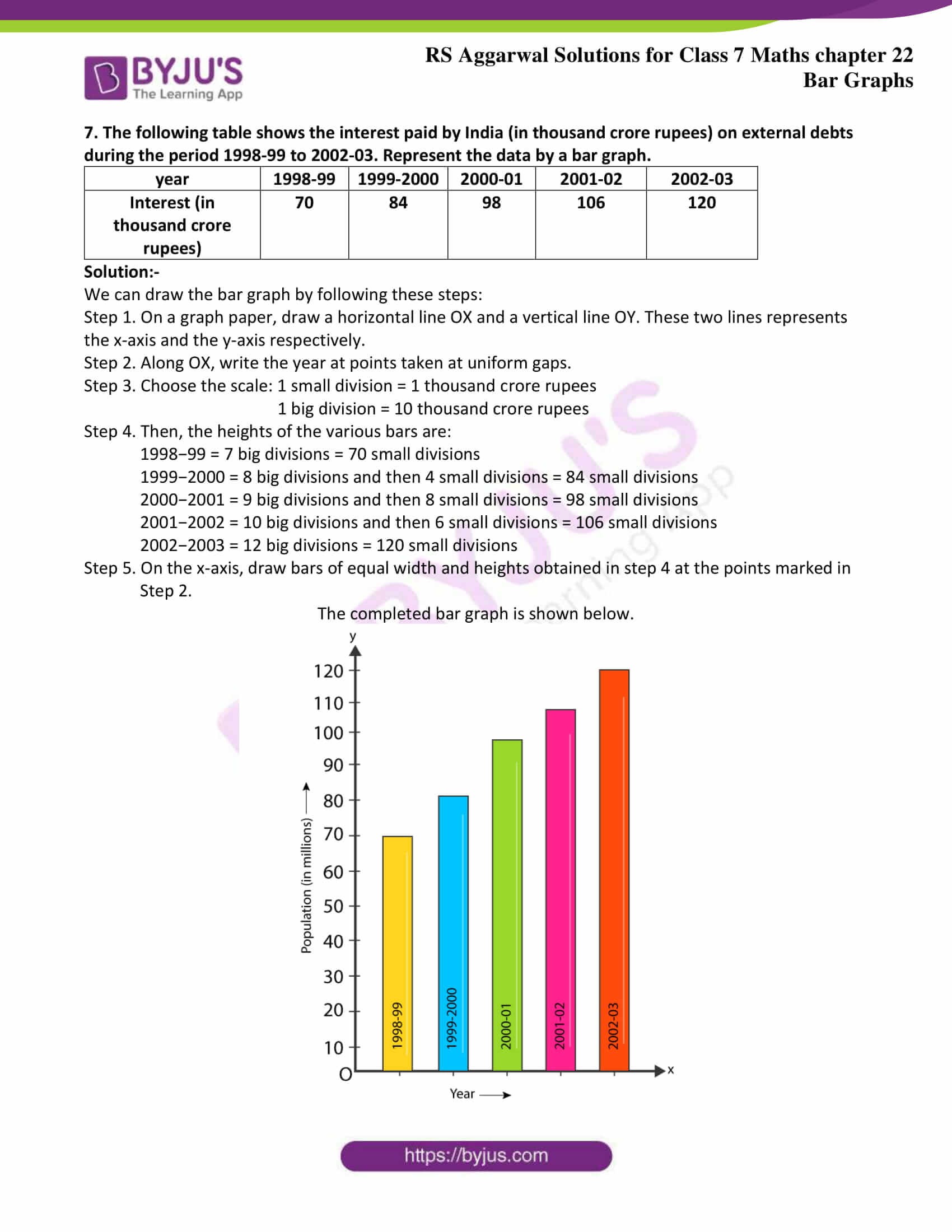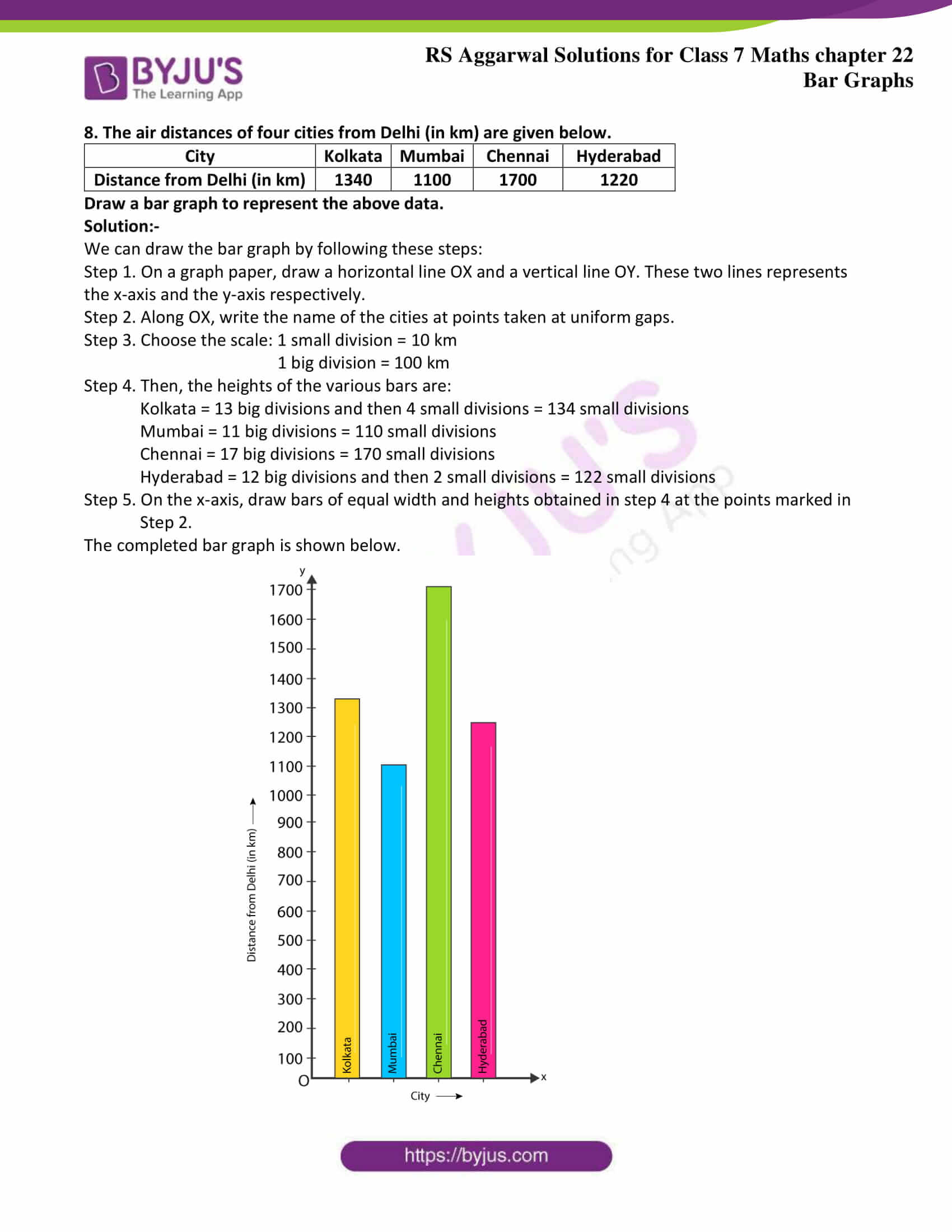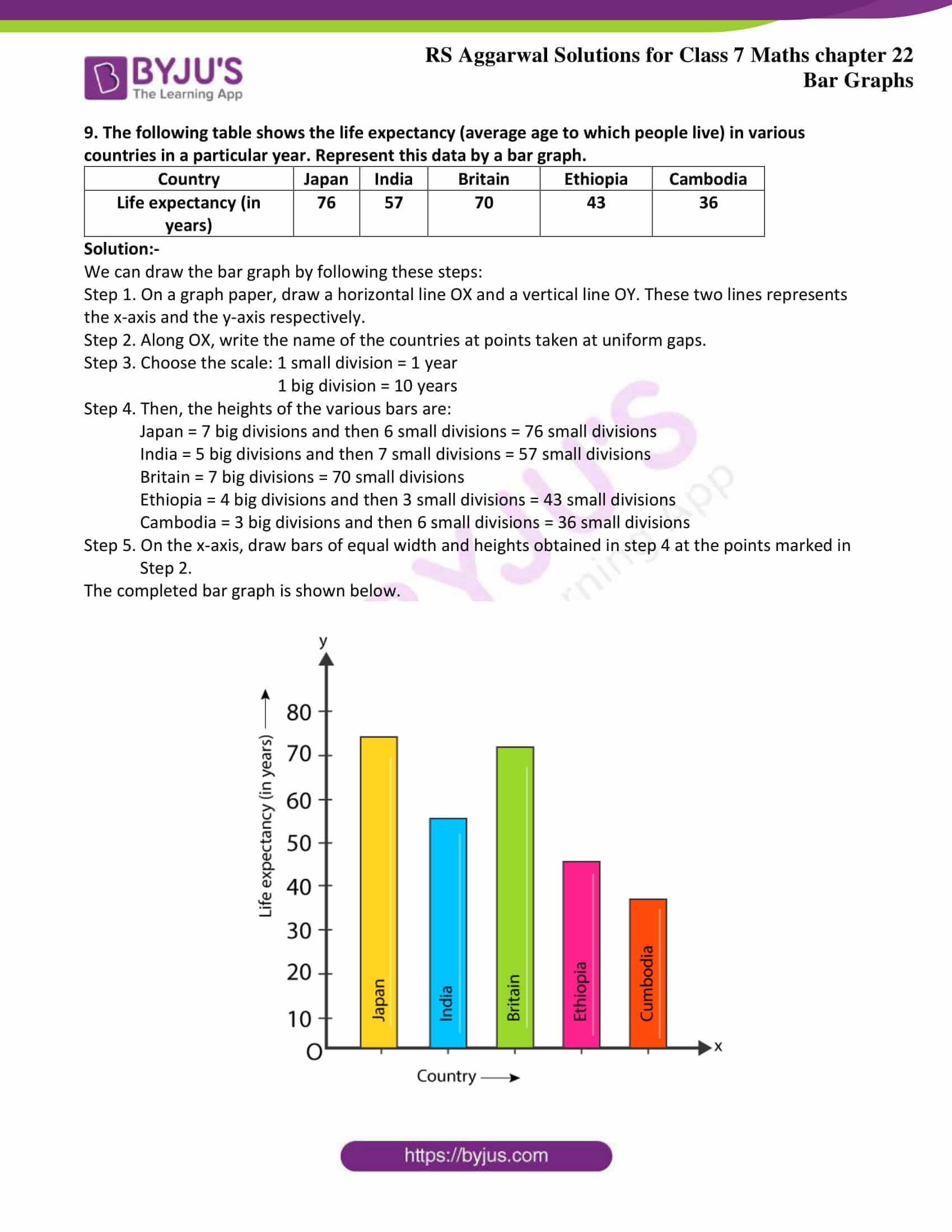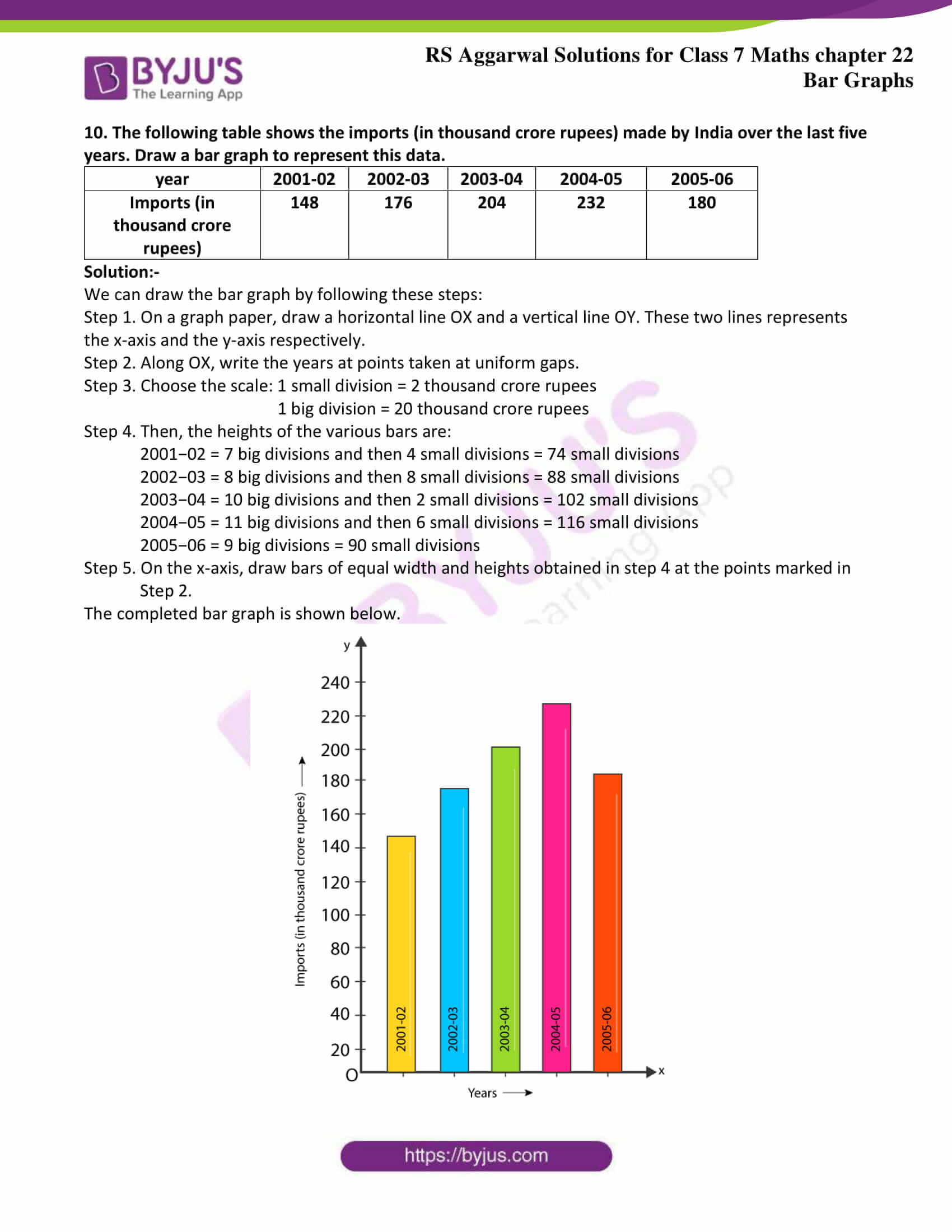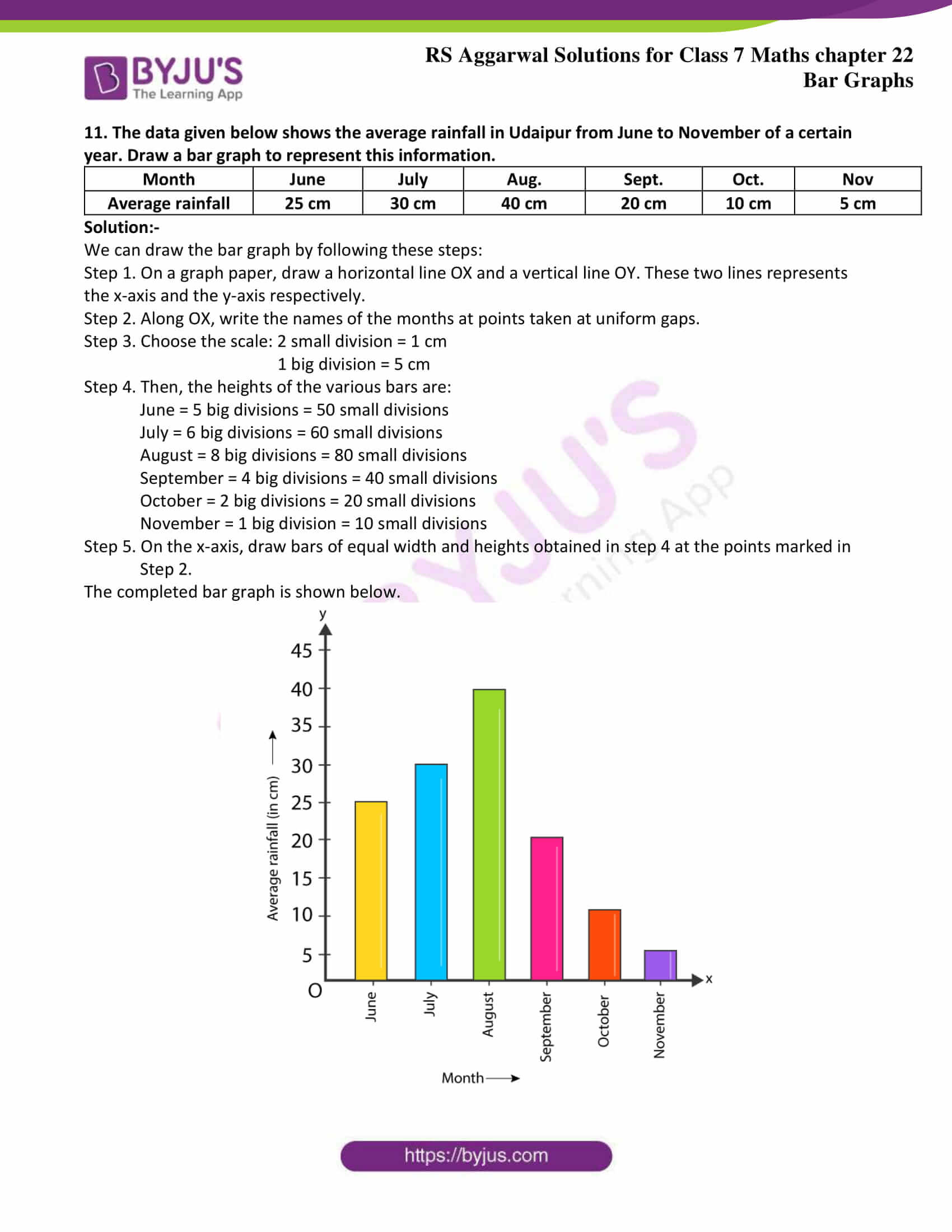## Exercise 22 Page: 276

1. The marks of a student in different subjects are given below.

 Subject Hindi English Maths Science Social Science Marks 43 56 80 65 50

Draw a bar graph from the above information.

Solution:-

We can draw the bar graph by following these steps:

Step 1. On a graph paper, draw a horizontal line OX and a vertical line OY. These two lines represents the x-axis and the y-axis respectively.

Step 2. Along OX, write the names of the subjects at points taken at uniform gaps.

Step 3. Choose the scale: 1 small division = 1 mark

1 big division = 10 marks

Step 4. Then, the heights of the various bars are:

Hindi = 4 big divisions and then 3 small divisions = 43 small divisions

English = 5 big divisions and then 6 small divisions = 56 small divisions

Maths = 8 big divisions = 80 small divisions

Science = 6 big divisions and 5 small divisions = 65 small divisions

Social Science = 5 big divisions = 50 small divisions

Step 5. On the x-axis, draw bars of equal width and heights obtained in step 4 at the points marked in

Step 2.

The completed bar graph is shown below.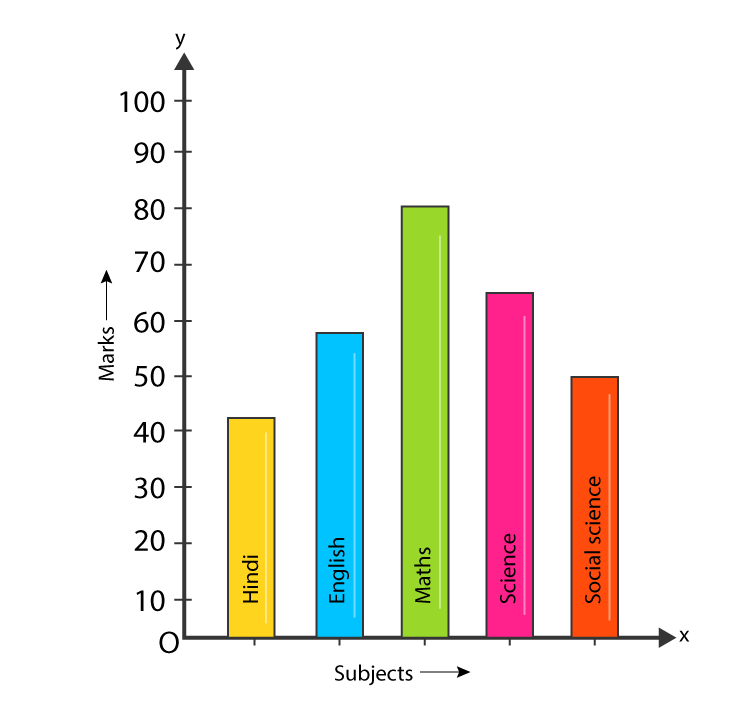2. The following table shows the favorite sports of 250 students of a school.
Represent the data by a bar graph.

 Sports Cricket Football Tennis Badminton Swimming No. of Students 75 35 50 25 65

Solution:-

We can draw the bar graph by following these steps:

Step 1. On a graph paper, draw a horizontal line OX and a vertical line OY. These two lines represents the x-axis and the y-axis respectively.

Step 2. Along OX, write the names of the sports at points taken at uniform gaps.

Step 3. Choose the scale: 1 small division = 1 student

1 big division = 10 students

Step 4. Then, the heights of the various bars are:

Cricket = 7 big divisions and then 5 small divisions = 75 small divisions

Football = 3 big divisions and then 5 small divisions = 35 small divisions

Tennis = 5 big divisions = 50 small divisions

Badminton = 2 big divisions and then 5 small divisions = 25 small divisions

Swimming = 6 big divisions and then 5 small divisions = 65 small divisions

Step 5. On the x-axis, draw bars of equal width and heights obtained in step 4 at the points marked in

Step 2.

The completed bar graph is shown below.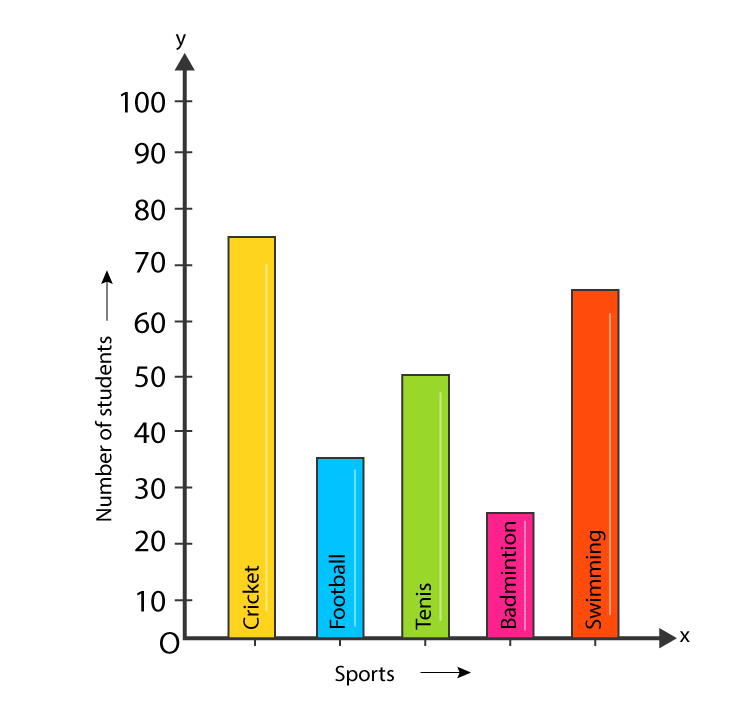3. Given below is a table which shows the year wise strength of a school. Represent this data by a bar graph.

 Year 2011 – 12 2012 – 13 2013 – 14 2014 – 15 2015 -16 No. of Students 800 975 1100 1400 1625

Solution:-

We can draw the bar graph by following these steps:

Step 1. On a graph paper, draw a horizontal line OX and a vertical line OY. These two lines represents the x-axis and the y-axis respectively.

Step 2. Along OX, write the years at points taken at uniform gaps.

Step 3. Choose the scale: 1 small division = 10 students

1 big division = 100 students

Step 4. Then, the heights of the various bars are:

2011-2012 = 8 big divisions = 80 small divisions

2012-2013 = 9 big divisions and then 7.5 small divisions = 97.5 small divisions

2013-2014 = 11 big divisions = 110 small divisions

2014-2015 = 14 big divisions = 140 small divisions

2015-2016 = 16 big divisions and then 2.5 small divisions = 162.5 small divisions

Step 5. On the x-axis, draw bars of equal width and heights obtained in step 4 at the points marked in

Step 2.

The completed bar graph is shown below.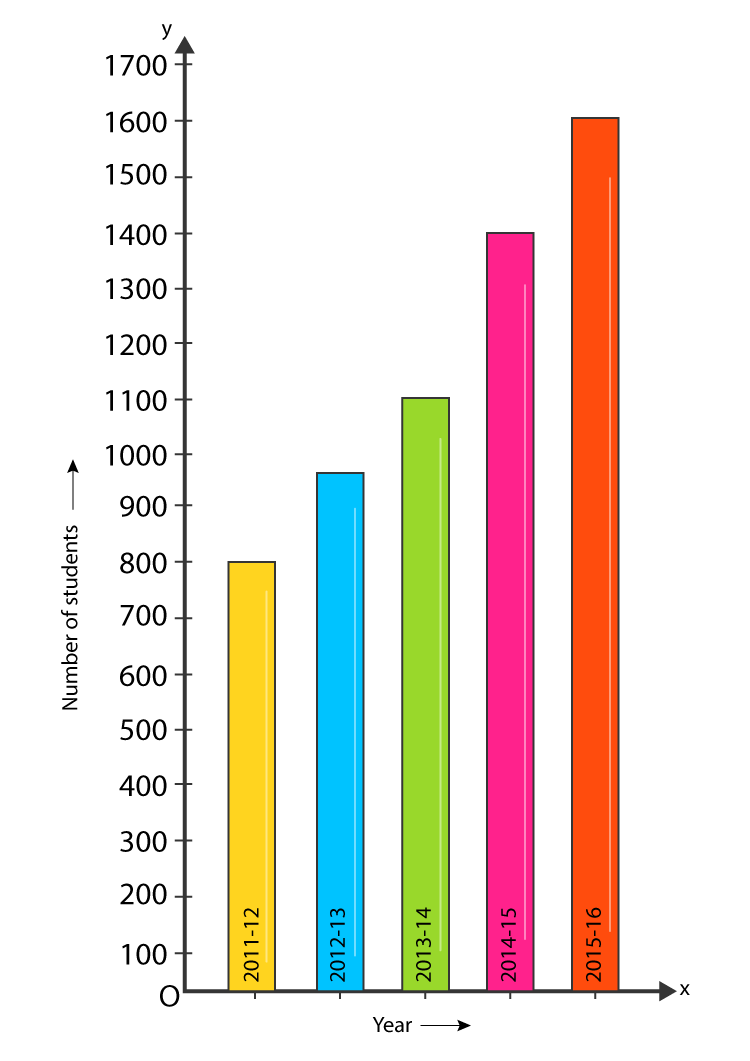4. The following table shows the number of scooters produced by a company during six consecutive years. Draw a bar graph to represent this data.

 Year 2011 2012 2013 2014 2015 2016 No. of Scooters 11000 14000 12500 17500 15000 24000

Solution:-

We can draw the bar graph by following these steps:

Step 1. On a graph paper, draw a horizontal line OX and a vertical line OY. These two lines represents the x-axis and the y-axis respectively.

Step 2. Along OX, write the years at points taken at uniform gaps.

Step 3. Choose the scale: 1 small division = 200 scooters

1 big division = 2000 scooters

Step 4. Then, the heights of the various bars are:

2011 = 5 big divisions and then 5 small divisions = 55 small divisions

2012 = 7 big divisions = 70 small divisions

2013 = 6 big divisions and then 2.5 small divisions = 62.5 small divisions

2014 = 8 big divisions and then 7.5 small divisions = 87.5 small divisions

2015 = 7 big divisions and then 5 small divisions = 75 small divisions

2016 = 12 big divisions = 120 small divisions

Step 5. On the x-axis, draw bars of equal width and heights obtained in step 4 at the points marked in

Step 2.

The completed bar graph is shown below.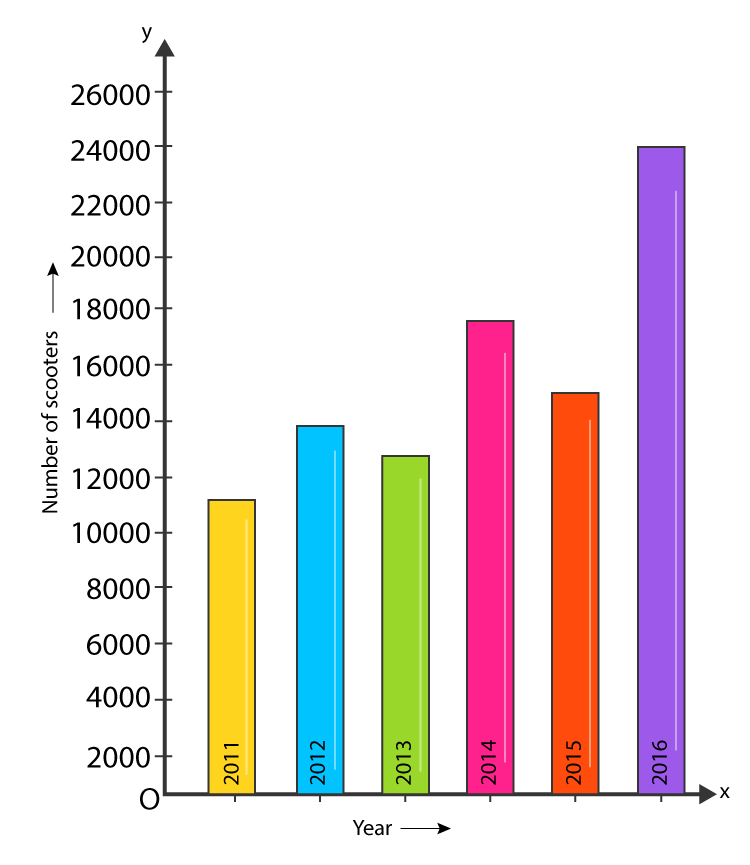5. The birth rate per thousand in five countries over a period of time is shown below.

 Country China India Germany UK Sweden Birth rate per thousand 42 35 14 28 21

Represent the above data by a bar graph.

Solution:-

We can draw the bar graph by following these steps:

Step 1. On a graph paper, draw a horizontal line OX and a vertical line OY. These two lines represents the x-axis and the y-axis respectively.

Step 2. Along OX, write the names of the country at points taken at uniform gaps.

Step 3. Choose the scale: 2 small division = 1 unit

1 big division = 5 units

Step 4. Then, the heights of the various bars are:

China = 8 big divisions and then 4 small divisions = 84 small divisions

India = 7 big divisions = 70 small divisions

Germany = 2 big divisions and then 8 small divisions = 28 small divisions

U.K. = 5 big divisions and then 6 small divisions = 56 small divisions

Sweden = 4 big divisions and then 2 small divisions = 42 small divisions

Step 5. On the x-axis, draw bars of equal width and heights obtained in step 4 at the points marked in

Step 2.

The completed bar graph is shown below.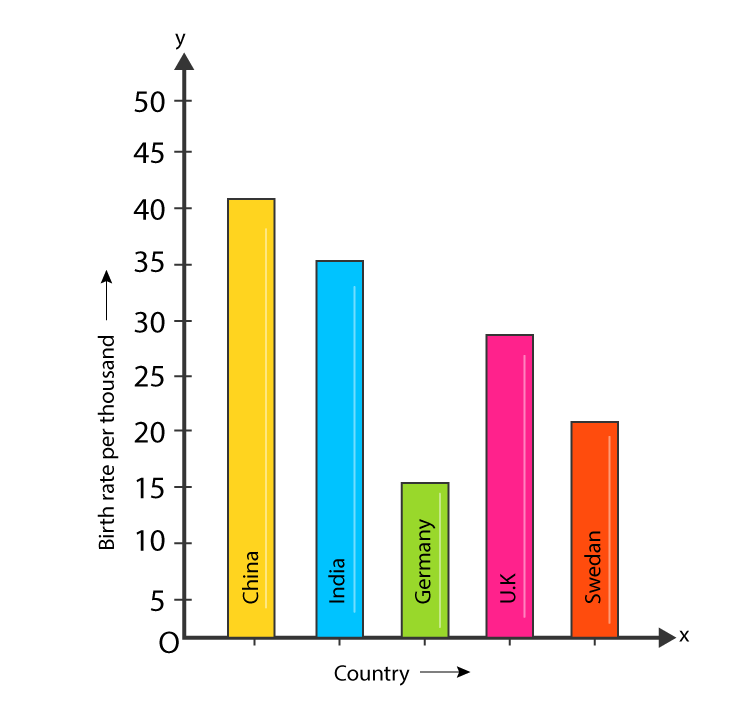6. The following data shows India’s total population (in millions) from 1961 to 2011.

 year 1961 1971 1981 1991 2001 2011 Population (in millions) 360 420 540 680 1020 1200

Solution:-

We can draw the bar graph by following these steps:

Step 1. On a graph paper, draw a horizontal line OX and a vertical line OY. These two lines represents the x-axis and the y-axis respectively.

Step 2. Along OX, write the year at points taken at uniform gaps.

Step 3. Choose the scale: 1 small division = 20 millions

1 big division = 200 millions

Step 4. Then, the heights of the various bars are:

1961 = 1 big divisions and then 8 small divisions = 18 small divisions

1971 = 2 big divisions and then 1 small division = 21 small divisions

1981 = 2 big divisions and then 7 small divisions = 27 small divisions

1991 = 3 big divisions and then 4 small divisions = 34 small divisions

2001 = 5 big divisions and then 1 small divisions = 51 small divisions

2011 = 6 big divisions = 60 small divisions

Step 5. On the x-axis, draw bars of equal width and heights obtained in step 4 at the points marked in

Step 2.

The completed bar graph is shown below.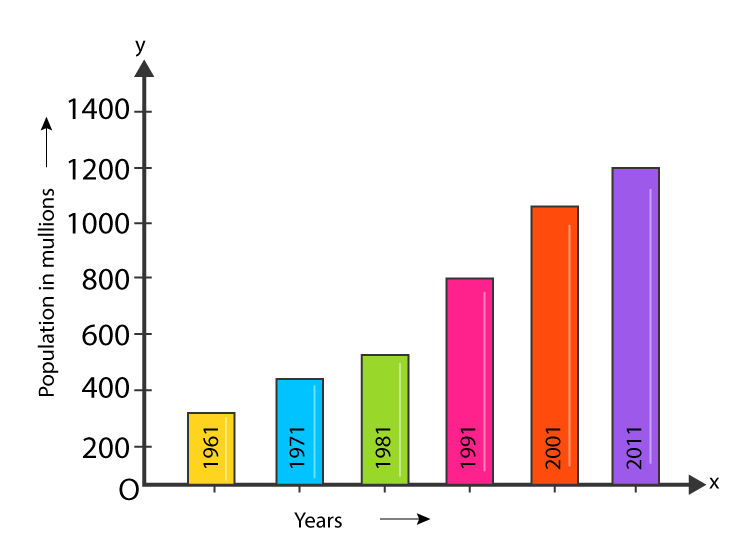7. The following table shows the interest paid by India (in thousand crore rupees) on external debts during the period 1998-99 to 2002-03. Represent the data by a bar graph.

 year 1998-99 1999-2000 2000-01 2001-02 2002-03 Interest (in thousand crore rupees) 70 84 98 106 120

Solution:-

We can draw the bar graph by following these steps:

Step 1. On a graph paper, draw a horizontal line OX and a vertical line OY. These two lines represents the x-axis and the y-axis respectively.

Step 2. Along OX, write the year at points taken at uniform gaps.

Step 3. Choose the scale: 1 small division = 1 thousand crore rupees

1 big division = 10 thousand crore rupees

Step 4. Then, the heights of the various bars are:

1998−99 = 7 big divisions = 70 small divisions

1999−2000 = 8 big divisions and then 4 small divisions = 84 small divisions

2000−2001 = 9 big divisions and then 8 small divisions = 98 small divisions

2001−2002 = 10 big divisions and then 6 small divisions = 106 small divisions

2002−2003 = 12 big divisions = 120 small divisions

Step 5. On the x-axis, draw bars of equal width and heights obtained in step 4 at the points marked in

Step 2.

The completed bar graph is shown below.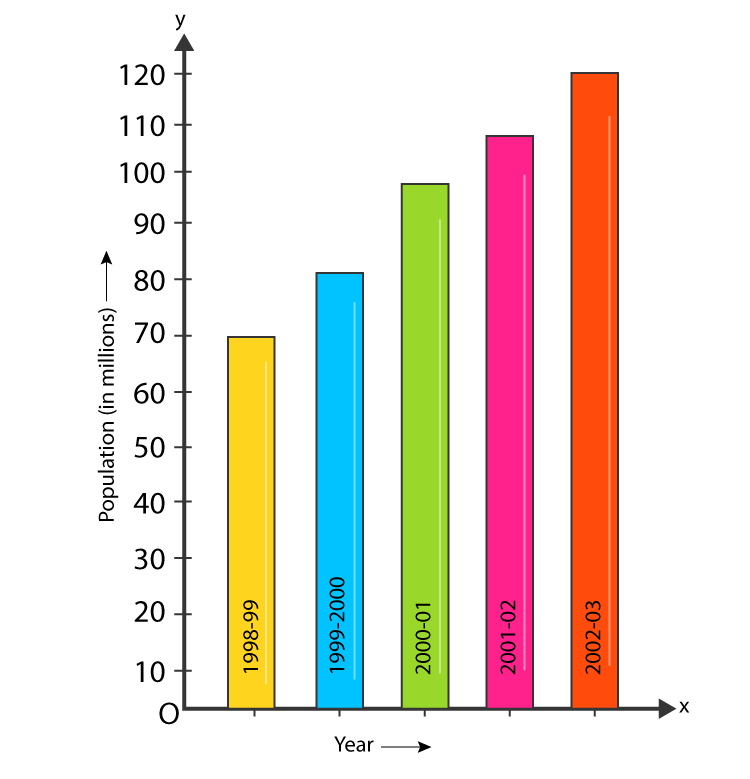8. The air distances of four cities from Delhi (in km) are given below.

 City Kolkata Mumbai Chennai Hyderabad Distance from Delhi (in km) 1340 1100 1700 1220

Draw a bar graph to represent the above data.

Solution:-

We can draw the bar graph by following these steps:

Step 1. On a graph paper, draw a horizontal line OX and a vertical line OY. These two lines represents the x-axis and the y-axis respectively.

Step 2. Along OX, write the name of the cities at points taken at uniform gaps.

Step 3. Choose the scale: 1 small division = 10 km

1 big division = 100 km

Step 4. Then, the heights of the various bars are:

Kolkata = 13 big divisions and then 4 small divisions = 134 small divisions

Mumbai = 11 big divisions = 110 small divisions

Chennai = 17 big divisions = 170 small divisions

Hyderabad = 12 big divisions and then 2 small divisions = 122 small divisions

Step 5. On the x-axis, draw bars of equal width and heights obtained in step 4 at the points marked in

Step 2.

The completed bar graph is shown below.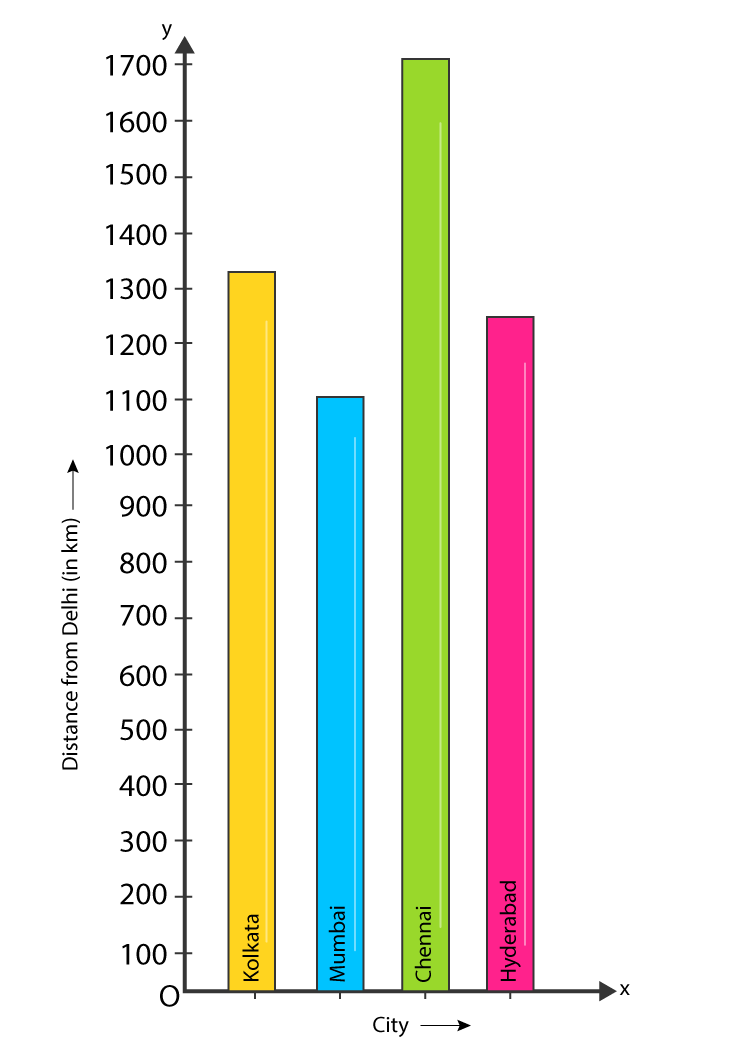9. The following table shows the life expectancy (average age to which people live) in various countries in a particular year. Represent this data by a bar graph.

 Country Japan India Britain Ethiopia Cambodia Life expectancy (in years) 76 57 70 43 36

Solution:-

We can draw the bar graph by following these steps:

Step 1. On a graph paper, draw a horizontal line OX and a vertical line OY. These two lines represents the x-axis and the y-axis respectively.

Step 2. Along OX, write the name of the countries at points taken at uniform gaps.

Step 3. Choose the scale: 1 small division = 1 year

1 big division = 10 years

Step 4. Then, the heights of the various bars are:

Japan = 7 big divisions and then 6 small divisions = 76 small divisions

India = 5 big divisions and then 7 small divisions = 57 small divisions

Britain = 7 big divisions = 70 small divisions

Ethiopia = 4 big divisions and then 3 small divisions = 43 small divisions

Cambodia = 3 big divisions and then 6 small divisions = 36 small divisions

Step 5. On the x-axis, draw bars of equal width and heights obtained in step 4 at the points marked in

Step 2.

The completed bar graph is shown below.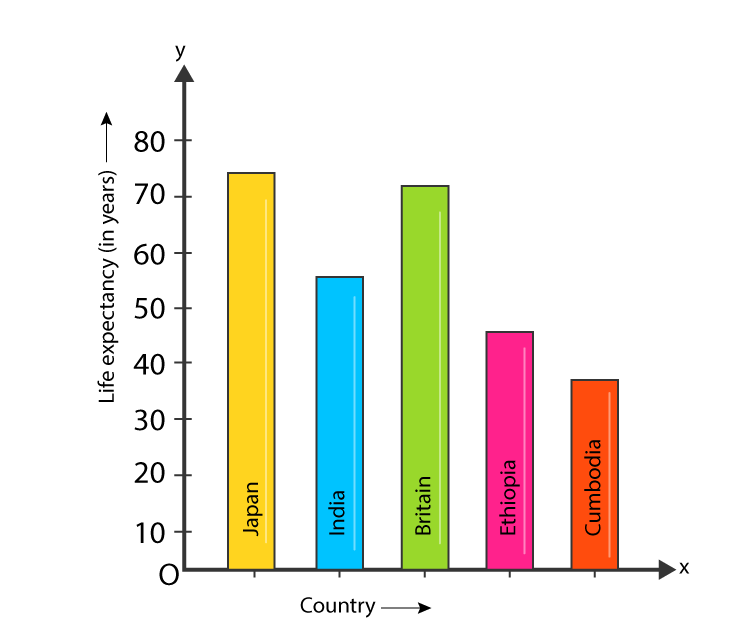10. The following table shows the imports (in thousand crore rupees) made by India over the last five years. Draw a bar graph to represent this data.

 year 2001-02 2002-03 2003-04 2004-05 2005-06 Imports (in thousand crore rupees) 148 176 204 232 180

Solution:-

We can draw the bar graph by following these steps:

Step 1. On a graph paper, draw a horizontal line OX and a vertical line OY. These two lines represents the x-axis and the y-axis respectively.

Step 2. Along OX, write the years at points taken at uniform gaps.

Step 3. Choose the scale: 1 small division = 2 thousand crore rupees

1 big division = 20 thousand crore rupees

Step 4. Then, the heights of the various bars are:

2001−02 = 7 big divisions and then 4 small divisions = 74 small divisions

2002−03 = 8 big divisions and then 8 small divisions = 88 small divisions

2003−04 = 10 big divisions and then 2 small divisions = 102 small divisions

2004−05 = 11 big divisions and then 6 small divisions = 116 small divisions

2005−06 = 9 big divisions = 90 small divisions

Step 5. On the x-axis, draw bars of equal width and heights obtained in step 4 at the points marked in

Step 2.

The completed bar graph is shown below.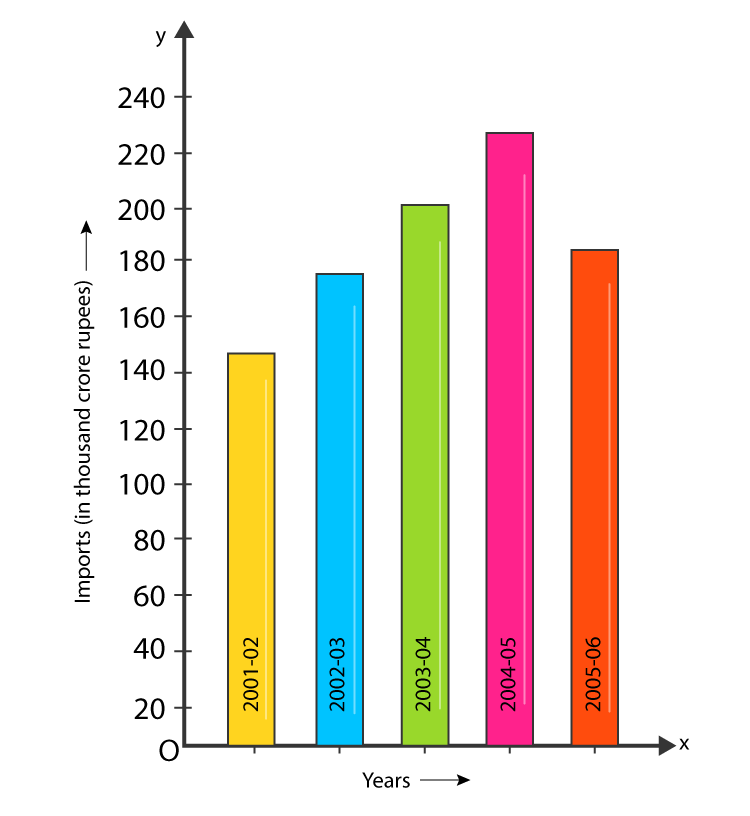11. The data given below shows the average rainfall in Udaipur from June to November of a certain year. Draw a bar graph to represent this information.

 Month June July Aug. Sept. Oct. Nov Average rainfall 25 cm 30 cm 40 cm 20 cm 10 cm 5 cm

Solution:-

We can draw the bar graph by following these steps:

Step 1. On a graph paper, draw a horizontal line OX and a vertical line OY. These two lines represents the x-axis and the y-axis respectively.

Step 2. Along OX, write the names of the months at points taken at uniform gaps.

Step 3. Choose the scale: 2 small division = 1 cm

1 big division = 5 cm

Step 4. Then, the heights of the various bars are:

June = 5 big divisions = 50 small divisions

July = 6 big divisions = 60 small divisions

August = 8 big divisions = 80 small divisions

September = 4 big divisions = 40 small divisions

October = 2 big divisions = 20 small divisions

November = 1 big division = 10 small divisions

Step 5. On the x-axis, draw bars of equal width and heights obtained in step 4 at the points marked in

Step 2.

The completed bar graph is shown below.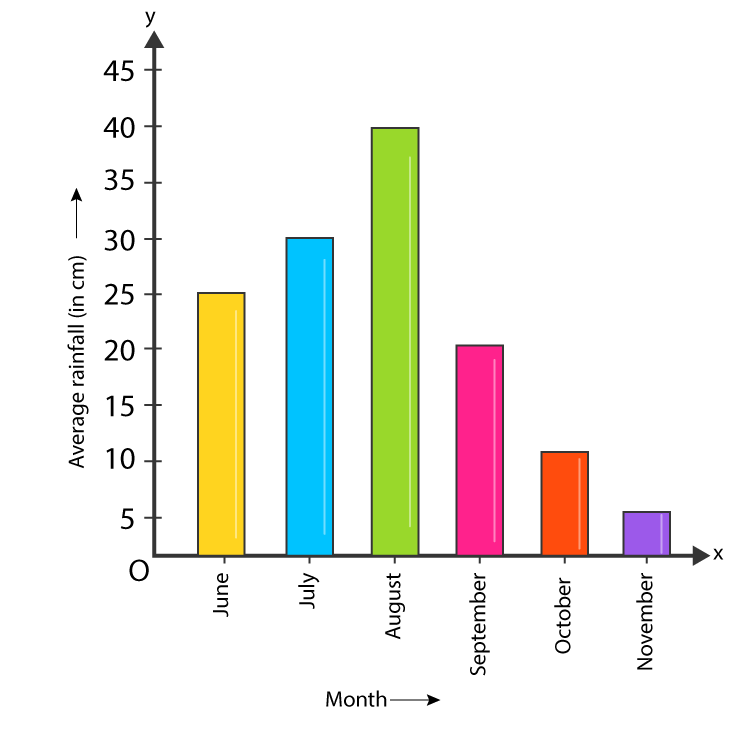## RS Aggarwal Solutions for Class 7 Maths Chapter 22 – Bar Graphs

Chapter 22 – Bar Graphs contains 1 exercise and the RS Aggarwal Solutions available on this page provide solutions to the questions present in the exercises. Now, let us have a look at some of the concepts discussed in this chapter.